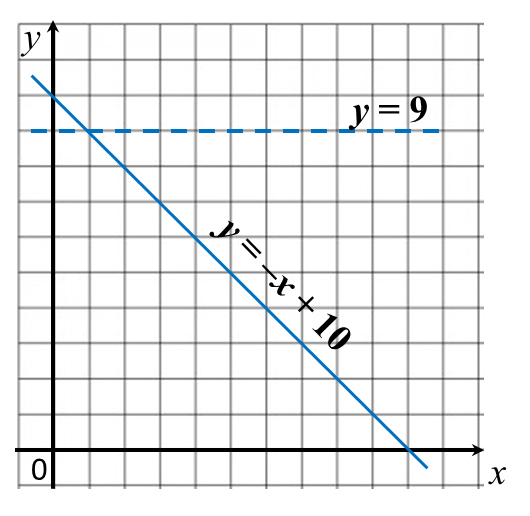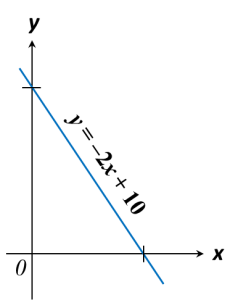# 2.3.1 Region Representing Inequalities in Two Variables (Examples)

Example 1:
On the graph in the answer space, shade the region which satisfies the three inequalities ≥ –x + 10, y > x and y < 9.Solution:
1.  The region that satisfies the inequality ≥ –x + 10 is the region on and above the line y = –x + 10.

2.  The region that satisfies the inequality > x is the region above the line y = x. Since inequality sign “ >” is used, the points on the line = x is not included. Thus, a dashed line needs to be drawn for y = x.

3.  The region that satisfies the inequality < 9 is the region below the line y = 9 drawn as a dashed line.Example 2:
On the graph in the answer space, shade the region which satisfies the three inequalities ≥ –2x + 10, y ≤ 10 and x < 5.Solution:
1. The region which satisfies the inequality of the form yax + c is the region that lies on and above the line y = ax+ c.

2. In this question, y intercept, c = 10, x intercept is 5.

3. The region that satisfies the inequality ≥ –2x + 10 is the region on and above the line y = –2x + 10.

4. The region that satisfies the inequality ≤ 10 is the region on and below the line y = 10.

5. The region that satisfies the inequality < 5 is on the left of the line x = 5 drawn as a dashed line.